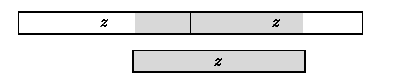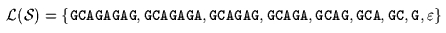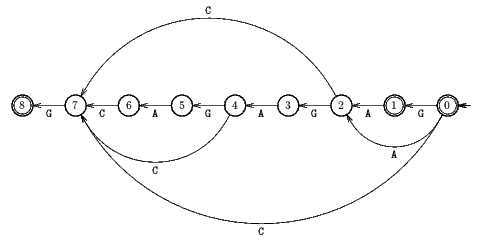Name
• String
• Substring
• Search
Edit

It is possible to make the Reverse Factor algorithm linear. It is in fact enough to remember the prefix u of x matched during the last attempt. Then during the current attempt when reaching the right end of u, it is easy to show that it is sufficient to read again at most the rightmost half of u. This is made by the Turbo Reverse Factor algorithm.

If a word z is a factor of a word w we define disp(z,w) the displacement of z in w to be the least integer d>0 such that w[m-d-|z|-1 .. m-d]=z.

The general situation of the Turbo Reverse Factor algorithm is when a prefix u is found in the text during the last attempt and for the current attempt the algorithm tries to match the factor v of length m-|u| in the text immediately at the right of u. If v is not a factor of x then the shift is computed as in the Reverse Factor algorithm. If v is a suffix of x then an occurrence of x has been found. If v is not a suffix but a factor of x then it is sufficient to scan again the min(per(u),|u|/2) rightmost characters of u. If u is periodic (i.e. per(u)|u|/2) let z be the suffix of u of length per(u). By definition of the period z is an acyclic word and then an overlap such as shown in Figure 23.1 is impossible.Figure 23.1: Impossible overlap if z is an acyclic word.

Thus z can only occur in u at distances multiple of per(u) which implies that the smallest proper suffix of uv which is a prefix of x has a length equal to |uv|-disp(zv,x)=m-disp(zv,x). Thus the length of the shift to perform is disp(zv,x).

If u is not periodic (per(u)>|u|/2), it is obvious that x can not reoccur in the left part of u of length per(u). It is then sufficient to scan the right part of u of length |u|-per(u) < |u|/2 to find a non defined transition in the automaton.

The function disp is implemented directly in the automaton S(x) without changing the complexity of its construction.

The preprocessing phase consists in building the suffix automaton of xR. It can be done in O(m) time complexity.

The searching phase is in O(n) time complexity. The Turbo Reverse Factor performs at most 2n inspections of text characters and it is also optimal in average performing On.log(m) / m )inspections of text characters on the average reaching the best bound shown by Yao in 1979.

Main features:

• refinement of the Reverse Factor algorithm;
• preprocessing phase in O(m) time and space complexity;
• searching phase in O(n) time complexity;
• performs 2n text characters inspections in the worst case;
• optimal in the average.

Example:

Preprocessing phaseSuffix automaton used by Turbo Reverse Factor algorithm.

Searching phase

 G C A T C G C A G A G A G T A T A C A G T A C G * 8 7 2 G C A G A G A G

Shift by: 5 (8 - 3)

 G C A T C G C A G A G A G T A T A C A G T A C G - - - 5 4 3 2 1 G C A G A G A G

Shift by: 7 (8 - 1)

 G C A T C G C A G A G A G T A T A C A G T A C G * 7 2 1 G C A G A G A G

Shift by: 7 (8 - 1)

The Turbo Reverse Factor algorithm performs 13 character comparisons on the example.

C